Question

# CJ10 18 P.009 Two spherical objects are separated by a distance that is 5.40 x 103...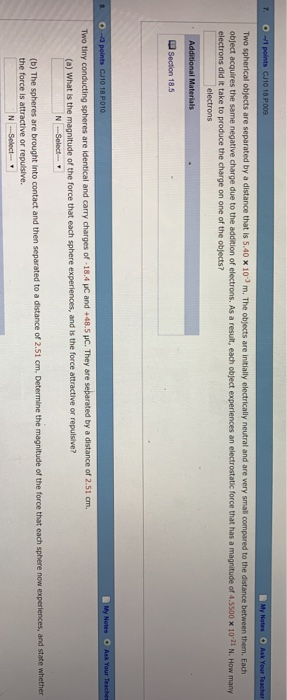CJ10 18 P.009 Two spherical objects are separated by a distance that is 5.40 x 103 m. The objects are initially electrically neutral and are very small o ect acquires the same negative charge due to the addition of electrons. As a result, each object experiences an electrostatic force that has a magnitude of 4 SS00 x 10-21 N How many electrons did it take to produce the charge on one of the objects? electrons CJ10 18 P010 Two tinyc ng spheres are identical and carry charges of -18.4 HC and +48.5 HC. They are separated by a distance of 2.51 cm. (o) What is the magnitude of the force that each sphere experiences, and is the force atactive or repuisive? (b) The spheres are brought into contact and then separated to a distance of 2.51 cm the force is attractive or repulsive. Determine the magnitude of the force that each sphere now experiences, and state whether t NSelect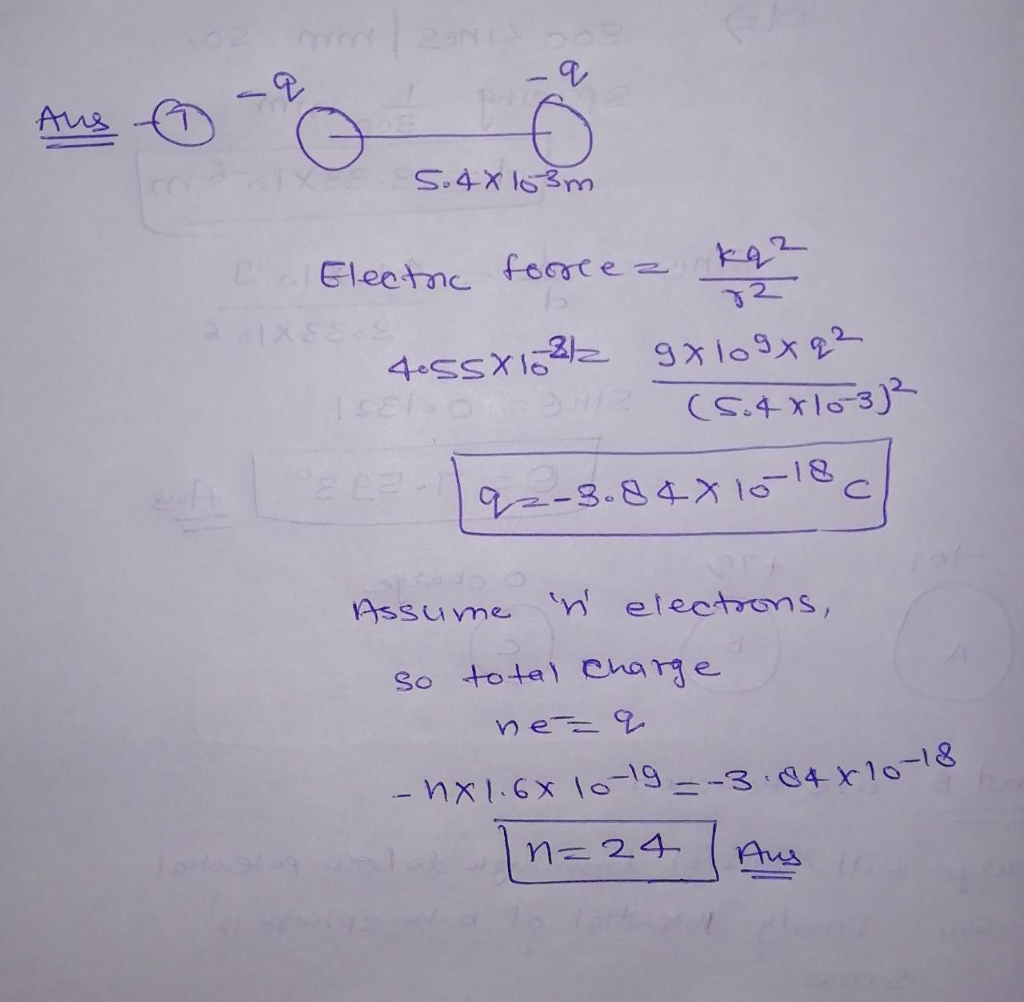#### Earn Coins

Coins can be redeemed for fabulous gifts.

Similar Homework Help Questions
• ### Do each question separately and show step by step Two spherical objects are separated by a...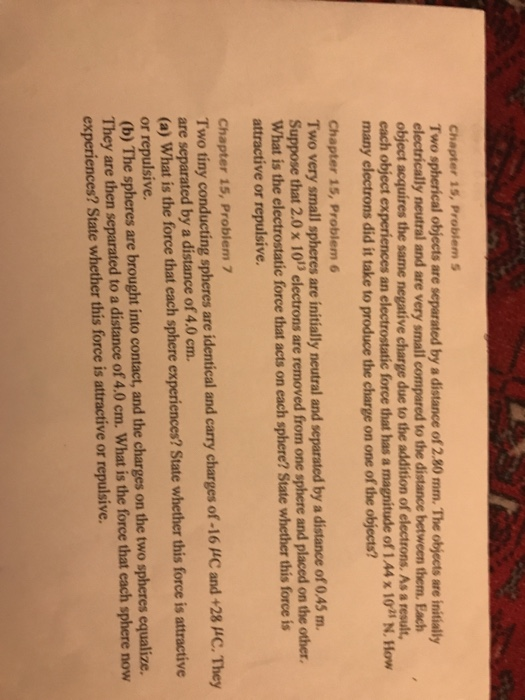Do each question separately and show step by step Two spherical objects are separated by a distance of 2.80 mm. The objects are initially electrically neutral and are very small compared to the distance between them. Each object acquires the same negative charge due to the addition of electrons, As a result, each object experiences an electrostatic force that has a magnitude of 1.44 times 10^-25 N. How many electrons did it take to produce the charge on one of...

• ### 18-10th Set A 0/1.5 points CJ10 18P In a vacuum, two particles have charges of q1...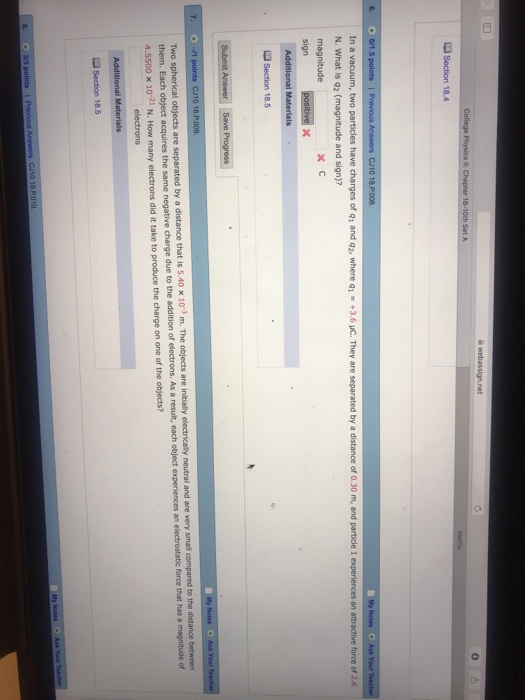18-10th Set A 0/1.5 points CJ10 18P In a vacuum, two particles have charges of q1 and q2, where q1 +3.6 uC. They are separated by a distance of 0.30 m, and particle 1 experiences an attractive force of 2.6 N. What is q2 (magnitude and sign)? magnitude sign L Section 18.5 7. -1 points CJ10 18 P.0059 Two spherical objects are separated by a distance that them. Each object acquires the same negative charge due to the addition of...

• ### My Notes O Ask Your Teacher -3 points CU10 18.P010 (a) What is the magnitude of...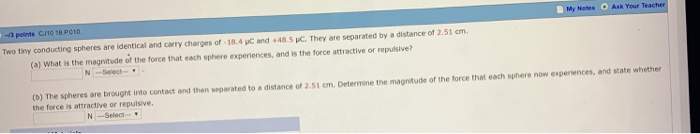My Notes O Ask Your Teacher -3 points CU10 18.P010 (a) What is the magnitude of the force that each sphere experiences, and is the force attractive or repulsive? N Select (b) The spheres are brought into contact and then separated to a distance of 2.51 cm. Determine the magnitude of the force that each sphere now experiences, and state whether the force is attractive or repuisive. N -Selec

• ### My Notes Ask Your Teacher 3. -/1 points CJ10 18.P.006.GO. A plate carries a charge of...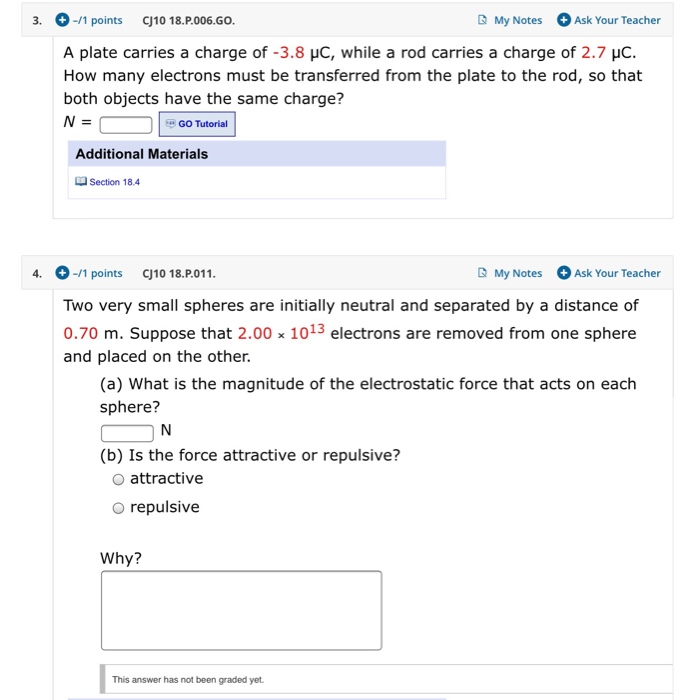My Notes Ask Your Teacher 3. -/1 points CJ10 18.P.006.GO. A plate carries a charge of -3.8 uC, while a rod carries a charge of 2.7 uc How many electrons must be transferred from the plate to the rod, so that both objects have the same charge? GO Tutorial N = Additional Materials Section 18.4 My Notes -/1 points CJ10 18.P.011 4. Ask Your Teacher Two very small spheres are initially neutral and separated by a distance of 0.70 m....

• ### Chapter 18, Problem 09 Two spherical objects are separated by a distance of 1.55 x 103...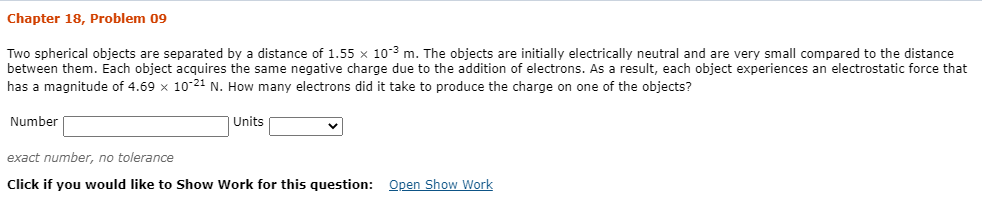Chapter 18, Problem 09 Two spherical objects are separated by a distance of 1.55 x 103 m. The objects are initially electrically neutral and are very small compared to the distance between them. Each object acquires the same negative charge due to the addition of electrons. As a result, each object experiences an electrostatic force that has a magnitude of 4.69 10-21 N. How many electrons did it take to produce the charge on one of the objects? Number Units...

• ### Two spherical objects are separated by a distance of 1.07 x 102m. The objects are initially...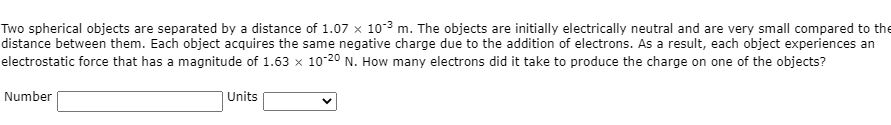Two spherical objects are separated by a distance of 1.07 x 102m. The objects are initially electrically neutral and are very small compared to the distance between them. Each object acquires the same negative charge due to the addition of electrons. As a result, each object experiences an electrostatic force that has a magnitude of 1.63 x 10-20 N. How many electrons did it take to produce the charge on one of the objects? Number Units

• ### Two spherical objects are separated by a distance that is 9.00 10-3 m. The objects are...

Two spherical objects are separated by a distance that is 9.00 10-3 m. The objects are initially electrically neutral and are very small compared to the distance between them. Each object acquires the same negative charge due to the addition of electrons. As a result, each object experiences an electrostatic force that has a magnitude of 1.1375 10-21 N. How many electrons did it take to produce the charge on one of the objects? electrons?

• ### Two spherical objects are separated by a distance of 1.25 × 10-3 m. The objects are...

Two spherical objects are separated by a distance of 1.25 × 10-3 m. The objects are initially electrically neutral and are very small compared to the distance between them. Each object acquires the same negative charge due to the addition of electrons. As a result, each object experiences an electrostatic force that has a magnitude of 1.78 × 10-20 N. How many electrons did it take to produce the charge on one of the objects?

• ### Two spherical objects are separated by a distance of 1.84 × 10-3 m. The objects are...

Two spherical objects are separated by a distance of 1.84 × 10-3 m. The objects are initially electrically neutral and are very small compared to the distance between them. Each object acquires the same negative charge due to the addition of electrons. As a result, each object experiences an electrostatic force that has a magnitude of 8.23 × 10-21 N. How many electrons did it take to produce the charge on one of the objects?

• ### Two spherical objects are separated by a distance of 2.04 × 10-3 m. The objects are...

Two spherical objects are separated by a distance of 2.04 × 10-3 m. The objects are initially electrically neutral and are very small compared to the distance between them. Each object acquires the same negative charge due to the addition of electrons. As a result, each object experiences an electrostatic force that has a magnitude of 1.08 × 10-20 N. How many electrons did it take to produce the charge on one of the objects?​